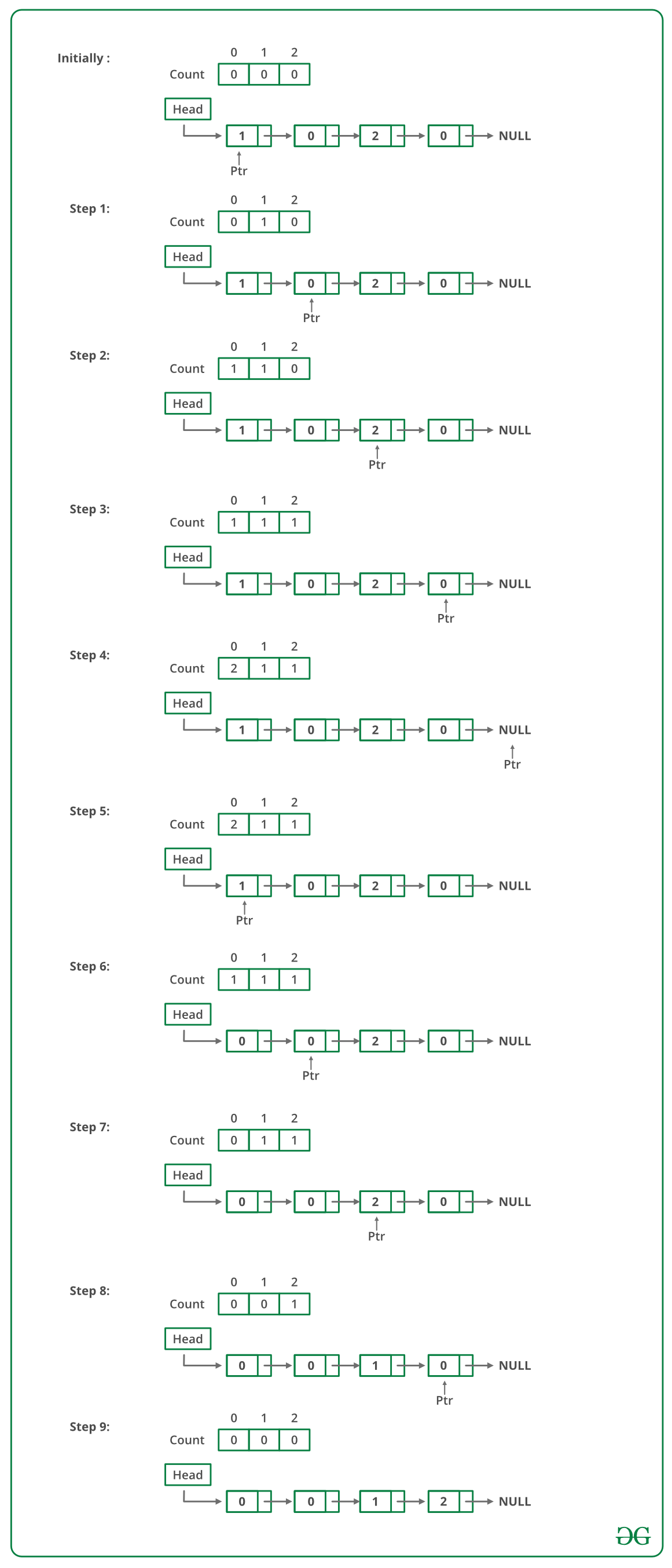# C++ Program For Sorting A Linked List Of 0s, 1s And 2s

• Last Updated : 22 Dec, 2021

Given a linked list of 0s, 1s and 2s, sort it.
Examples:

```Input: 1 -> 1 -> 2 -> 0 -> 2 -> 0 -> 1 -> NULL
Output: 0 -> 0 -> 1 -> 1 -> 1 -> 2 -> 2 -> NULL

Input: 1 -> 1 -> 2 -> 1 -> 0 -> NULL
Output: 0 -> 1 -> 1 -> 1 -> 2 -> NULL
```

Source: Microsoft Interview | Set 1

Following steps can be used to sort the given linked list.

• Traverse the list and count the number of 0s, 1s, and 2s. Let the counts be n1, n2, and n3 respectively.
• Traverse the list again, fill the first n1 nodes with 0, then n2 nodes with 1, and finally n3 nodes with 2.

Below image is a dry run of the above approach:Below is the implementation of the above approach:

## C++

 `// C++ Program to sort a linked list ``// 0s, 1s or 2s ``#include ``using` `namespace` `std;`` ` `// Link list node ``class` `Node ``{ ``    ``public``:``    ``int` `data; ``    ``Node* next; ``}; `` ` `// Function to sort a linked list ``// of 0s, 1s and 2s ``void` `sortList(Node *head) ``{ ``    ``// Initialize count of '0', '1' ``    ``// and '2' as 0 ``    ``int` `count = {0, 0, 0}; ``    ``Node *ptr = head; `` ` `    ``/* Count total number of '0', '1' and '2' ``       ``count will store total number of '0's ``       ``count will store total number of '1's ``       ``count will store total number of '2's */``    ``while` `(ptr != NULL) ``    ``{ ``        ``count[ptr->data] += 1; ``        ``ptr = ptr->next; ``    ``} `` ` `    ``int` `i = 0; ``    ``ptr = head; `` ` `    ``/* Let say count = n1, count = n2 ``       ``and count = n3.``       ``Now start traversing list from head node, ``       ``1) fill the list with 0, till n1 > 0 ``       ``2) fill the list with 1, till n2 > 0 ``       ``3) fill the list with 2, till n3 > 0 */``    ``while` `(ptr != NULL) ``    ``{ ``        ``if` `(count[i] == 0) ``            ``++i; ``        ``else``        ``{ ``            ``ptr->data = i; ``            ``--count[i]; ``            ``ptr = ptr->next; ``        ``} ``    ``} ``} `` ` `// Function to push a node``void` `push (Node** head_ref, ``           ``int` `new_data) ``{ ``    ``// Allocate node ``    ``Node* new_node = ``new` `Node();`` ` `    ``// Put in the data ``    ``new_node->data = new_data; `` ` `    ``// Link the old list off the ``    ``// new node ``    ``new_node->next = (*head_ref); `` ` `    ``// Move the head to point to the ``    ``// new node ``    ``(*head_ref) = new_node; ``} `` ` `// Function to print linked list ``void` `printList(Node *node) ``{ ``    ``while` `(node != NULL) ``    ``{ ``        ``cout << node->data << ``" "``; ``        ``node = node->next; ``    ``} ``    ``cout << endl; ``} `` ` `// Driver code``int` `main(``void``) ``{ ``    ``Node *head = NULL; ``    ``push(&head, 0); ``    ``push(&head, 1); ``    ``push(&head, 0); ``    ``push(&head, 2); ``    ``push(&head, 1); ``    ``push(&head, 1); ``    ``push(&head, 2); ``    ``push(&head, 1); ``    ``push(&head, 2); `` ` `    ``cout << ``"Linked List Before Sorting"``; ``    ``printList(head); `` ` `    ``sortList(head); `` ` `    ``cout << ``"Linked List After Sorting"``; ``    ``printList(head); `` ` `    ``return` `0; ``} ``// This code is contributed by rathbhupendra`

Output:

```Linked List Before Sorting
2  1  2  1  1  2  0  1  0